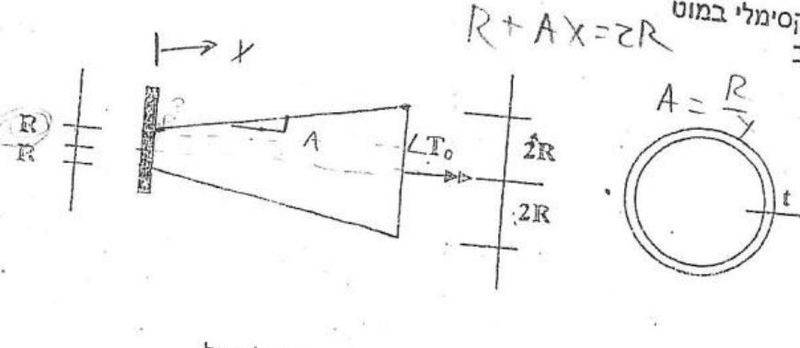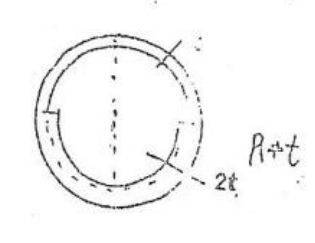# Finding stress in non symetric cross section

in a given shaft with a circular cross section, the radius changes linearlyfind the maximum shear stress

i used T*r/J
and since J is dependant on r^4, i found that the cross section with the smallest radius will feel the largest stress.

find the angle of twist at the end of the shaft

T/(GJ)*dx while J is a function of X and i integrate from 0 to L

if the bottom of the shaft is reinforced, -thickened- to 2t, what is the largest shear stress felt in the shaft??up till now i have only solved questions with either circular/ rectangular closed cross sections, or other open cross sections

can i use the equation for maximum stress
T/(2A*t)

where A is the area surrounded by an axis through the center of the side of the shape?? if so how do i do this ? what would that axis look like? would it be 2 half circles with 90 degree joints? meaning the area would be pi/2*(R12+R22) where R1 and R2 are the average radii of the 2 half circles??

using this logic i would find the maximum stress in the thin walled circle

is this correct? can i do this?

this is where i am stumped,